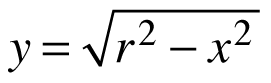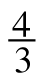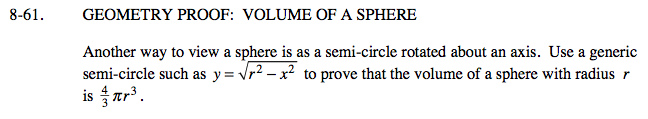### Home > CALC > Chapter 8 > Lesson 8.1.6 > Problem8-61

8-61.
1. GEOMETRY PROOF: VOLUME OF A SPHERE

2. Another way to view a sphere is as a semi-circle rotated about an axis. Use a generic semi-circle such asto prove that the volume of a sphere with radius r isπ r3. Homework Help ✎Set up and evaluate an integral showing what happens when you rotate the arbitrary semicircle about the x-axis.

Note:
r = radius of the sphere, which is a constant.
y = rotating radii, which are not constant.